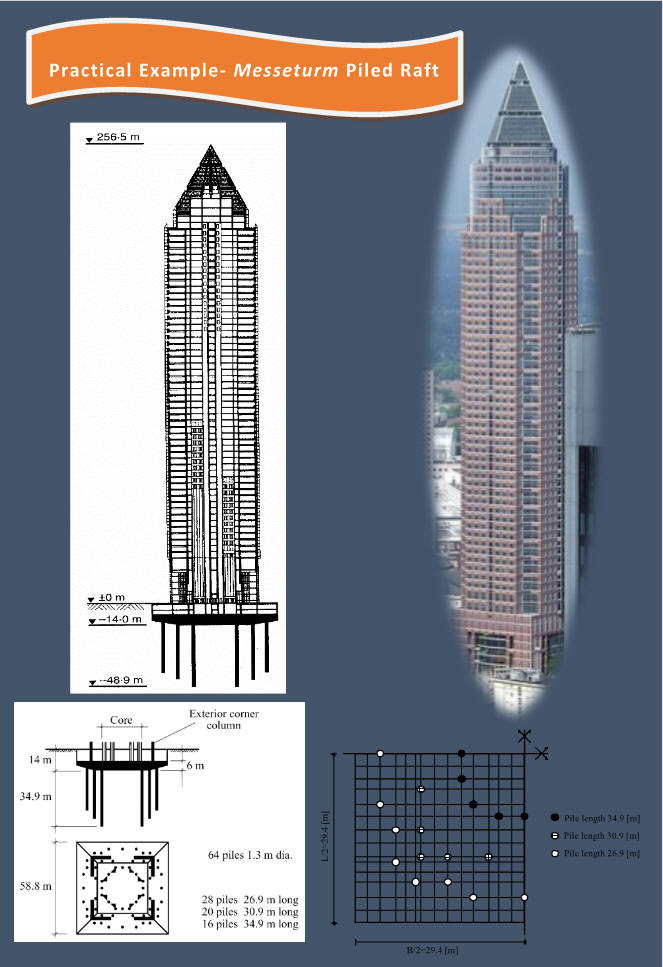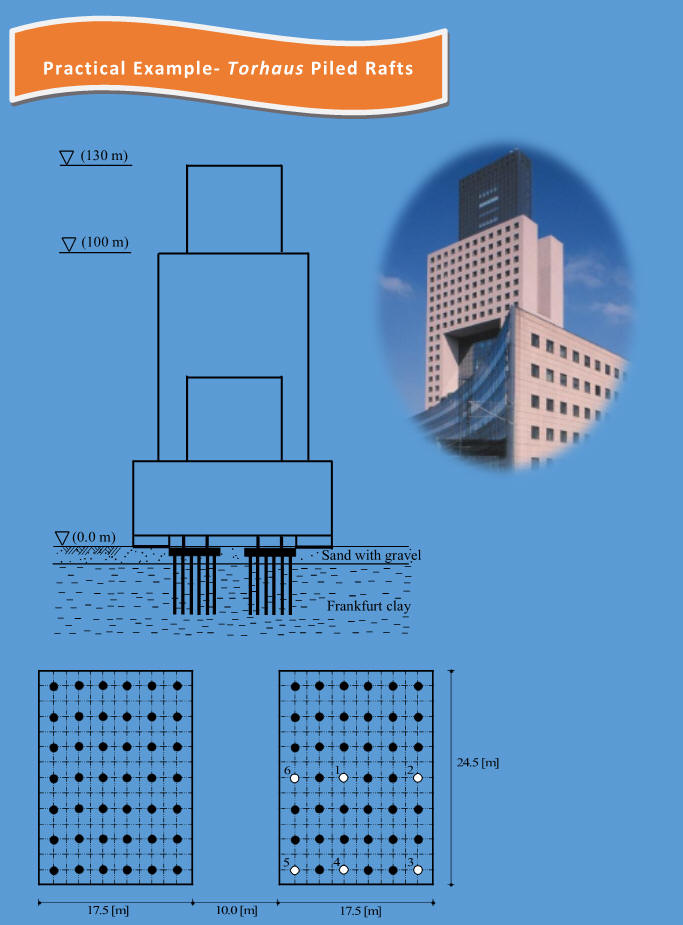## Piled Raft Foundation

ELPLA is a powerful tool for analyzing piled-raft foundations. ELPLA has models for analyzing single pile, pile groups, piled raft and friction piles in clay soil.Analysis of Piled Raft

Multiple Models to Meet Design Requirements

Practical Examples

Digital book and practical examples are provided with the software packageIn ELPLA, there are different calculation methods to analysis of the raft on piles. Therefore, the pile group for each method are required to define according to the used soil model as described in the next paragraphs.

Pile groups for Simple Assumption Model

In this model all forces acting on the raft will be transmit linearly on the piles. When the "Pile groups" command is chosen for this model, the following Table in Figure C-71 appears to define the pile diameter. Pile diameter is required for design of the slab for punching shear.Figure C-71  Defining pile groups for Simple Assumption Model

Pile groups for Winkler’s Model

For the two methods of Constant and Variable Modulus of Subgrade Reaction (methods 2 and 3), when the modulus of subgrade reaction is required to define by the user, pile groups in this case will be the pile diameter and the pile stiffness, Figure C-72.Figure C-72  Defining pile groups for Winkler’s Model

Pile groups for Isotropic Elastic Half-Space and Layered soil Models

When pile groups are required to define for one of these two soil models, the following Dialog box of Figure C-73 appears. The soil data around and under the pile are required to define. Soil data are used to determine the pile stiffness due to the soil type by ELPLA.Figure C-73  Defining pile groups for Half-Space and Layered soil Models